Question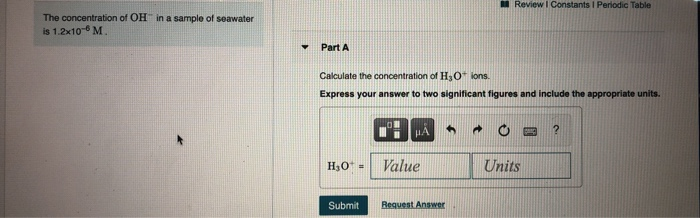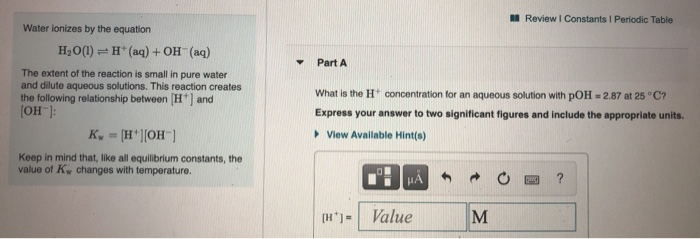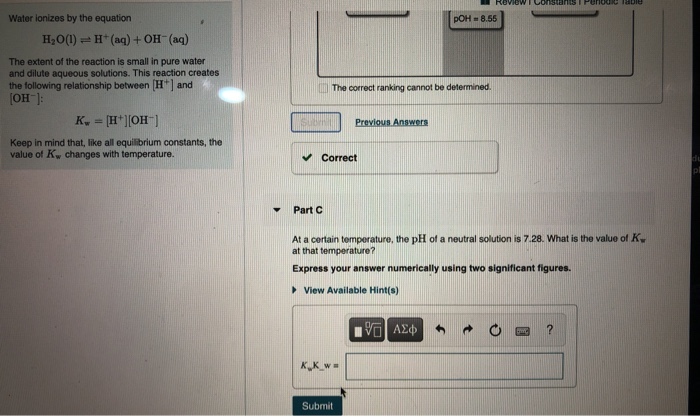We need at least 10 more requests to produce the answer.

0 / 10 have requested this problem solution

The more requests, the faster the answer.

All students who have requested the answer will be notified once they are available.

#### Earn Coins

Coins can be redeemed for fabulous gifts.

Similar Homework Help Questions
• ### Review | Constants Periodic Table Water ionizes by the equation H2 О(1) — н* (аq) + ОН (ад) The extent of the reaction...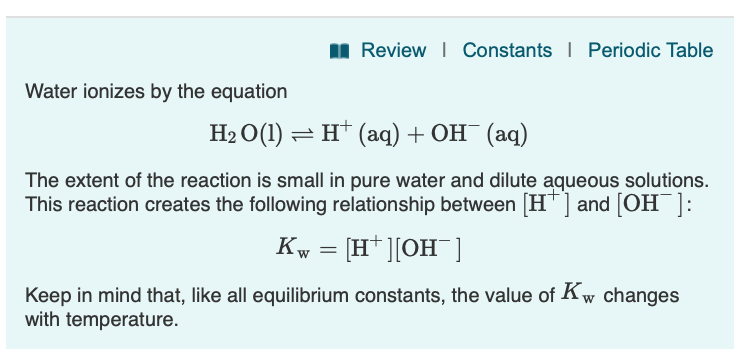Review | Constants Periodic Table Water ionizes by the equation H2 О(1) — н* (аq) + ОН (ад) The extent of the reaction is small in pure water and dilute aqueous solutions. This reaction creates the following relationship between [H ] and [OH ]: Kw 3D н'|Он ] Keep in mind that, like all equilibrium constants, the value of Kw changes with temperature Part C At a certain temperature, the pH of a neutral solution is 7.26. What is the...

• ### Water ionizes by the equation H2O(l)⇌H+(aq)+OH−(aq) The extent of the reaction is small in pure water...

Water ionizes by the equation H2O(l)⇌H+(aq)+OH−(aq) The extent of the reaction is small in pure water and dilute aqueous solutions. This reaction creates the following relationship between [H+] and [OH−]: Kw=[H+][OH−] Keep in mind that, like all equilibrium constants, the value of Kw changes with temperature. Part A What is the H+ concentration for an aqueous solution with pOH = 3.02 at 25 ∘C?

• ### Water ionizes by the equation H2 0()--H+ (aq) + OH-(aq) The extent of the reaction is...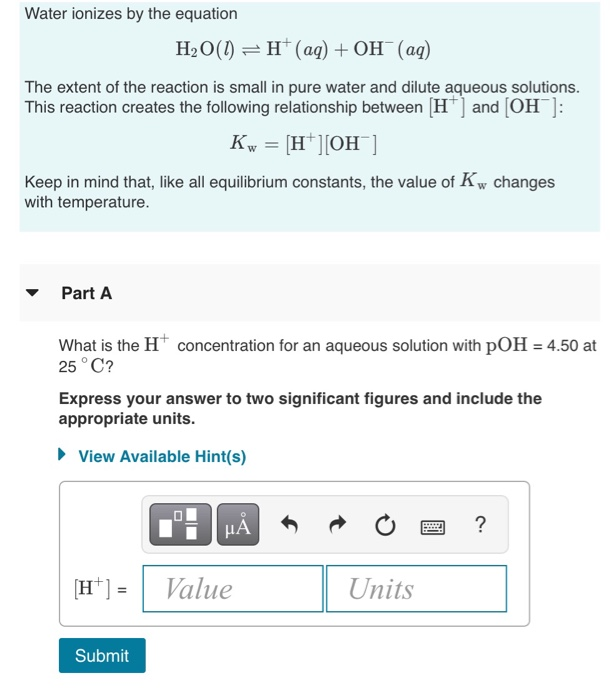Water ionizes by the equation H2 0()--H+ (aq) + OH-(aq) The extent of the reaction is small in pure water and dilute aqueous solutions. This reaction creates the following relationship between H and [OH Keep in mind that, like all equilibrium constants, the value of Kw changes with temperature. ▼ Part A What is the H concentration for an aqueous solution with pOH 4.50 at 25 ° C? Express your answer to two significant figures and include the appropriate units....

• ### Part A the value of Ky at that temperature? Water ionizes by the equation H2O(l) =...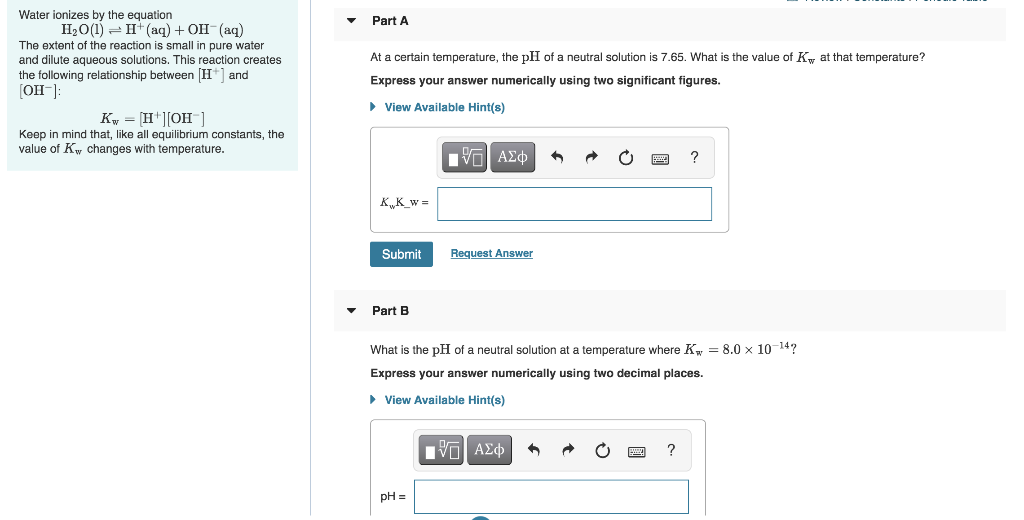Part A the value of Ky at that temperature? Water ionizes by the equation H2O(l) = 1+ (aq) + OH-(aq) The extent of the reaction is small in pure water and dilute aqueous solutions. This reaction creates the following relationship between (H+) and (OH): Ky = [H+][OH-] Keep in mind that, like all equilibrium constants, the value of Kw changes with temperature. At a certain temperature, the pH of a neutral solution is 7.65. What Express your answer numerically using...

• ### 1- Calculating [H+] for Pure Water: In a certain acidic solution at 25 ∘C, [H+] is...

1- Calculating [H+] for Pure Water: In a certain acidic solution at 25 ∘C, [H+] is 100 times greater than [OH −]. What is the value for [OH −] for the solution? In a certain acidic solution at 25 , [] is 100 times greater than [ ]. What is the value for [ ] for the solution? 1.0×10−8 M 1.0×10−7 M 1.0×10−6 M 1.0×10−2 M 1.0×10−9 M 2. ± Acid-Base Relationships in Water: Water ionizes by the equation H2O(l)⇌H+(aq)+OH−(aq)The...

• ### part b and c please ( 5 of 15 A Review | Constants Periodic Table Part...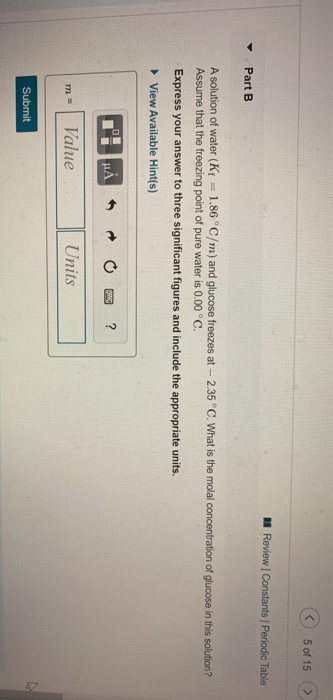part b and c please ( 5 of 15 A Review | Constants Periodic Table Part B A solution of water (Kf = 1.86°C/m) and glucose freezes at -- 2.35 °C. What is the molal concentration of glucose in this solution? Assume that the freezing point of pure water is 0.00 °C. Express your answer to three significant figures and include the appropriate units. View Available Hint(s) T'I HAO ? m= Value Units Submit A Review Constants Periodic Table AT)...

• ### NA Review | Constants 1 Periodic Table pH is a logarithmic scale used to indicate the...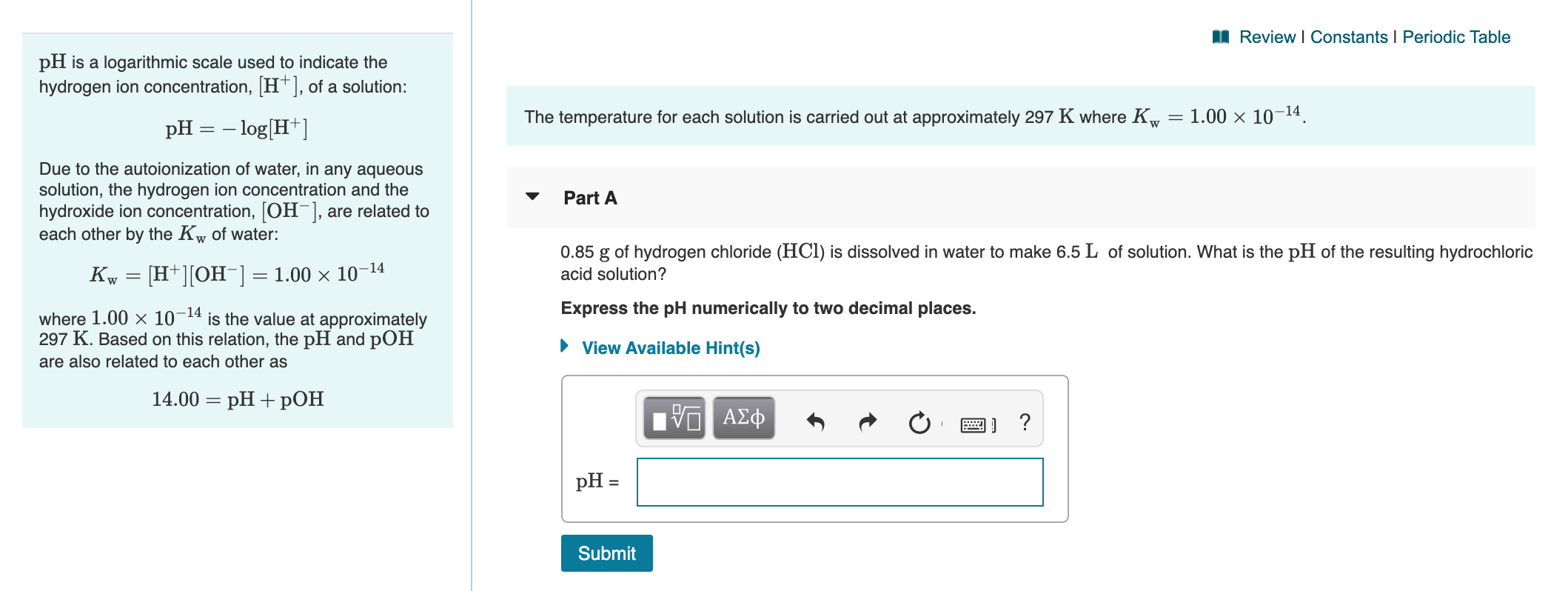NA Review | Constants 1 Periodic Table pH is a logarithmic scale used to indicate the hydrogen ion concentration, [H+], of a solution: The temperature for each solution is carried out at approximately 297 K where Kw = 1.00 x 10-14. pH = -log[H+] Due to the autoionization of water, in any aqueous solution, the hydrogen ion concentration and the hydroxide ion concentration, [OH-], are related to each other by the Kw of water: Part A Kw = [H+][OH-] =...

• ### Exercise 7.118 11 of 23 > Review Constants Periodic Table Part A Apure gold ning and...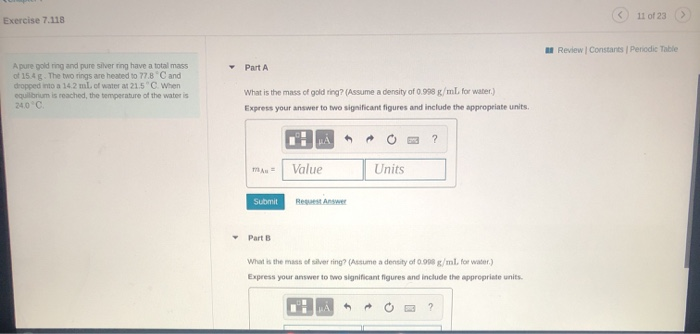Exercise 7.118 11 of 23 > Review Constants Periodic Table Part A Apure gold ning and pure silver ring have a total mass of 15.4g. The two rings are heated to 778 and dropped into a 14.2 ml.of water a 21.5 When equilibrium is reached, the temperature of the water is 2000 What is the mass of gold ring? (Assume a density of 0.998 g/ml for water.) Express your answer to two significant figures and include the appropriate units. mu...

• ### Review Constants Periodic Table You mix a 120.0-ml sample of a solution that is 0.0134 M...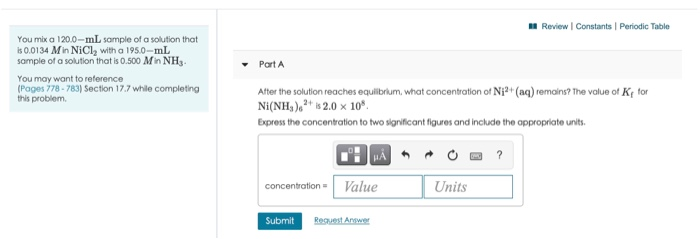Review Constants Periodic Table You mix a 120.0-ml sample of a solution that is 0.0134 M in NiCl, with a 195.0-ml sample of a solution that is 0.500 Min NH You may want to reference Pages 778 - 783) Section 17.7 while completing this problem Part A After the solution reaches equilibrium, what concentration of Ni2+ (aq) remains? The value of K for Ni(NH4)2 | 2.0 x 10*. Express the concentration to two significant figures and include the appropriate units....

• ### A Review Constants Periodic Table The changes in boiling point (AT) or freezing point (AT) in...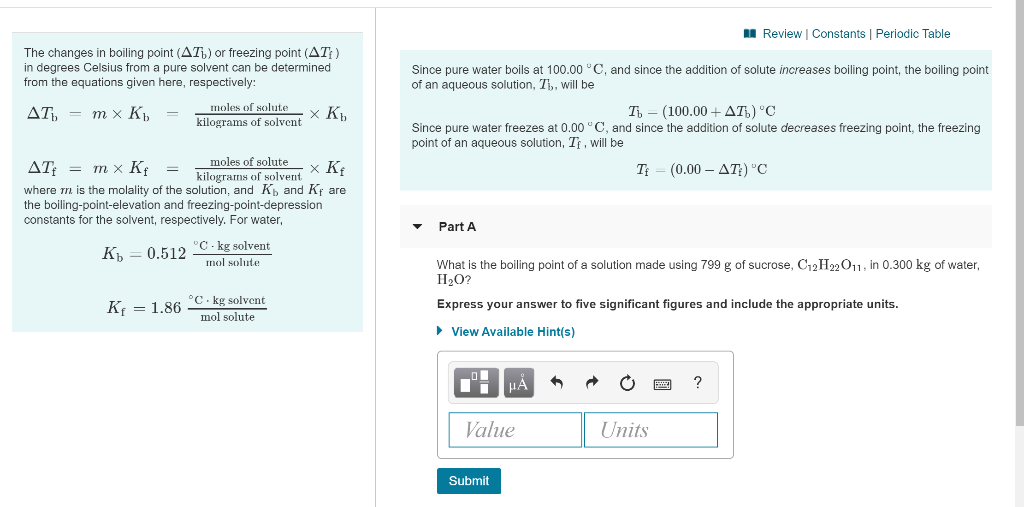A Review Constants Periodic Table The changes in boiling point (AT) or freezing point (AT) in degrees Celsius from a pure solvent can be determined from the equations given here, respectively: AT) = m x K = moles of solute XK K. kilograms of solvent Since pure water boils at 100.00 °C, and since the addition of solute increases boiling point, the boiling point of an aqueous solution, Th, will be T - (100.00+AT) 'C Since pure water freezes at...